Choose Language Hide Translation Bar
Highlighted

## How do I plot mathematical functions with JMP?

I realize that JMP isn't a mathematical function plotter, but suppose I have a function:  y=((v1+v2)*sin(v3+arcsine(v1/(v1+v2)))/sin(pi()-v3-arcsine(v1/(v1+v2))-v4/v1)-v1)

... and I'd like to plot it using Graph Builder.  Is there a convenient way to do this type of thing with JMP?  (preferably without resorting to JSL)

Seems like it would involve building a full-factorial matrix of inputs v1 thru v4, but it's not clear to me how to do that, even with the DOE FFD platform, especially if I'd like some of those variables to have hundreds of values, e.g., v1 to be 0 thru 5000 every 10.

1 ACCEPTED SOLUTION

Accepted Solutions
HighlightedJeff_PerkinsonCommunity Manager

## Re: How do I plot mathematical functions with JMP?

@BHarris wrote:
I understand -- I think I was mostly interested in knowing *how* to build the data table if I knew what levels I wanted for each of the inputs. Basically, is there an easy way to build a full-factorial-matrix data table given a min, increment, and max value for each variable?

Take a look at the Sequence() function (it's the Row functions in the Formula Editor),  and also Tables->Join, where you'll find a Cartesian join option.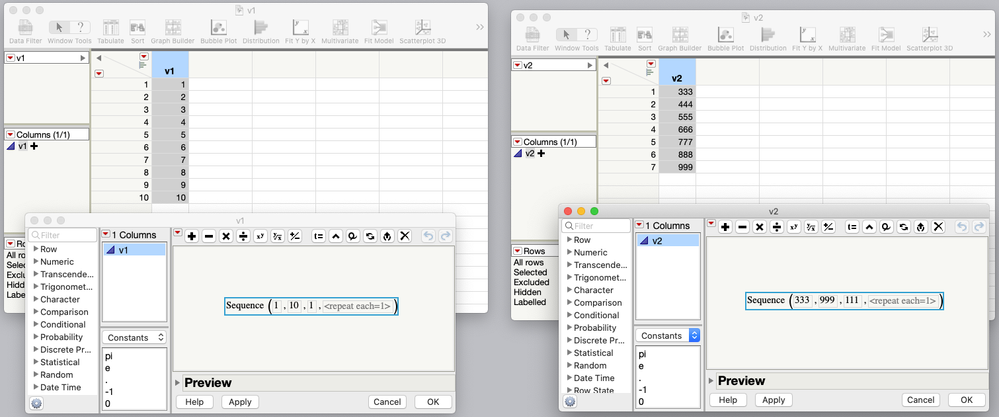Two data tables, one column each, Sequence() formula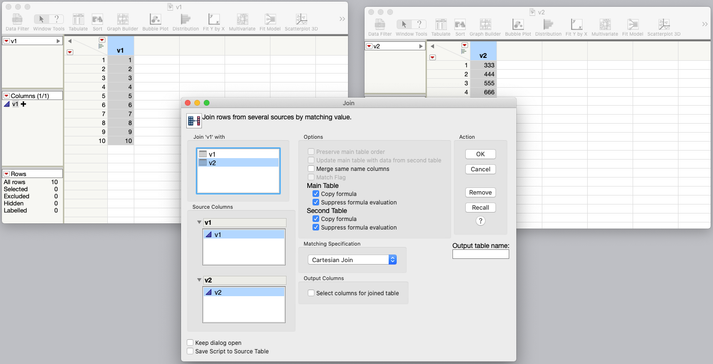Join Dialog, Cartesian Join option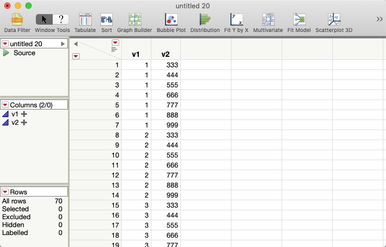Resulting data table

-Jeff
17 REPLIES 17
Highlighted

## Re: How do I plot mathematical functions with JMP?

The Nonlinear Model platform and the simulators work well for this. If I get time, I'll send an example.

Highlighted

## Re: How do I plot mathematical functions with JMP?

Surface Plot can do this too. With NO data tables open, start surface plot from the menu, then set up like this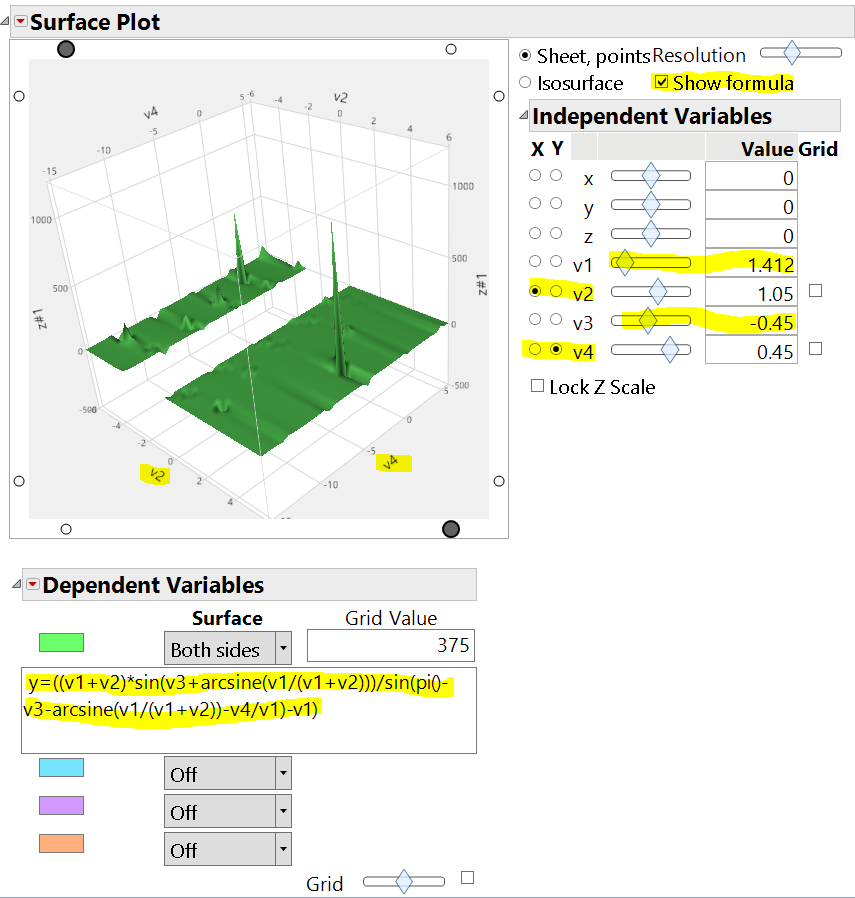Choose the vN for two of the axes and set values for the other vN

Show Formulas, replace the default formula with your own formula, pick your axis variables, use the mouse to rotate and adjust the axes. I'm pretty sure your formula generates missing values for some vN values, and the surface is being interrupted by them.

Craige
Highlightedstan_koprowskiCommunity Manager

## Re: How do I plot mathematical functions with JMP?

Cool.  I did not know that.

I learn something new everyday.

cheers,

Stan

Highlighted

## Re: How do I plot mathematical functions with JMP?

There's a little bit more, see Three JSL Easter EggsTriple Helix spinning in 3D

Craige
Highlighted

## Re: How do I plot mathematical functions with JMP?

How did you get JMP to auto-rotate the Triple-Helix?
Highlighted

## Re: How do I plot mathematical functions with JMP?

shift key with mouse drag or arrow keys. It saves the rotation angle/speed in the script and will come up already rotating.

Craige
Highlighted

## Re: How do I plot mathematical functions with JMP?

ain't that the truth!

Jim
Highlighted

## Re: How do I plot mathematical functions with JMP?

I had no idea JMP would do this, pretty amazing! Reminds me of the old Graphing Calculator app on the PowerPC Mac.

I asked about Graph Builder because we need to end up with a family of line plots (X, Y, group X, group Y, overlay) that we can read values from.

But I'm going to share this with the other JMP users at my work, super cool. Thanks!
Highlighted

## Re: How do I plot mathematical functions with JMP?

I do not think that Graph Builder will work, even though it can plot the graph of a function of one independent variable. The only roles available for a case like this is X and Y. You have four independent variables, so the plot is 5 dimensions.

Would the Profiler suffice? If so, make a new data table. Create a data column for V1-V4 and Y. You do not need a grid. Add two rows to the data table and enter the minimum and maximum value for each independent variable. Enter the formula for the function to the Y column.Then select Graph > Profiler and enter Y for the formula. You will likely have to manually adjust the Y axis in the profiler. The algorithm won't detect the cyclic extremes in your function well on its own.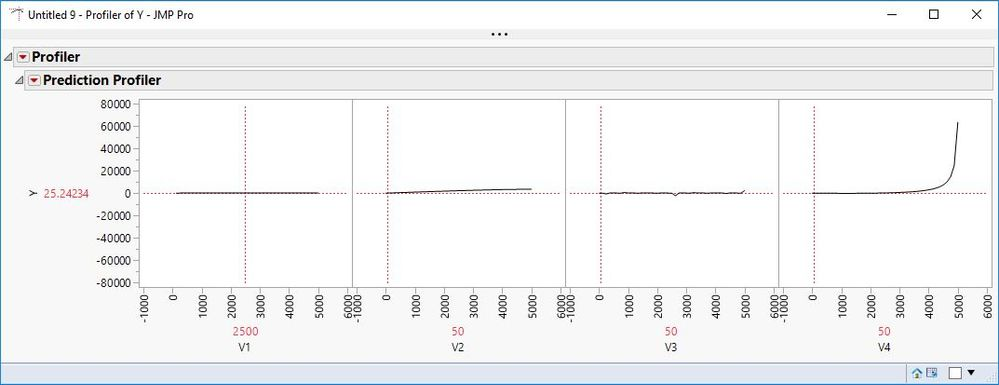I attached the data table for my set up and a table script to launch the profiler for you to play with.

Learn it once, use it forever!
Article Labels